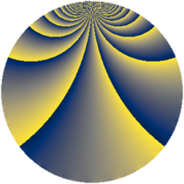# Properties

 Label 1183.2.bbLevel $1183$ Weight $2$ Character orbit 1183.bb Rep. character $\chi_{1183}(437,\cdot)$ Character field $\Q(\zeta_{12})$ Dimension $368$ Sturm bound $242$

# Learn more

## Defining parameters

 Level: $$N$$ $$=$$ $$1183 = 7 \cdot 13^{2}$$ Weight: $$k$$ $$=$$ $$2$$ Character orbit: $$[\chi]$$ $$=$$ 1183.bb (of order $$12$$ and degree $$4$$) Character conductor: $$\operatorname{cond}(\chi)$$ $$=$$ $$91$$ Character field: $$\Q(\zeta_{12})$$ Sturm bound: $$242$$

## Dimensions

The following table gives the dimensions of various subspaces of $$M_{2}(1183, [\chi])$$.

Total New Old
Modular forms 544 448 96
Cusp forms 432 368 64
Eisenstein series 112 80 32

## Trace form

 $$368q + 2q^{2} + 12q^{3} + 6q^{5} + 6q^{7} + 16q^{8} + 144q^{9} + O(q^{10})$$ $$368q + 2q^{2} + 12q^{3} + 6q^{5} + 6q^{7} + 16q^{8} + 144q^{9} + 10q^{11} - 80q^{14} + 44q^{15} + 116q^{16} + 4q^{18} - 12q^{19} + 26q^{21} + 12q^{24} + 6q^{28} - 32q^{29} - 24q^{31} - 4q^{32} - 48q^{33} - 52q^{35} + 8q^{37} + 60q^{40} + 12q^{42} + 42q^{44} + 24q^{45} - 12q^{46} - 30q^{47} - 88q^{50} - 20q^{53} - 78q^{54} - 40q^{57} - 26q^{58} + 54q^{59} - 16q^{60} + 84q^{61} - 24q^{63} - 144q^{66} - 16q^{67} + 84q^{68} - 50q^{70} + 36q^{71} - 22q^{72} - 66q^{73} - 12q^{74} + 12q^{79} - 138q^{80} - 8q^{81} + 58q^{84} + 84q^{85} - 42q^{86} - 192q^{87} + 60q^{89} - 72q^{92} - 6q^{93} + 84q^{94} + 42q^{96} + 86q^{98} + 24q^{99} + O(q^{100})$$

## Decomposition of $$S_{2}^{\mathrm{new}}(1183, [\chi])$$ into newform subspaces

The newforms in this space have not yet been added to the LMFDB.

## Decomposition of $$S_{2}^{\mathrm{old}}(1183, [\chi])$$ into lower level spaces

$$S_{2}^{\mathrm{old}}(1183, [\chi]) \cong$$ $$S_{2}^{\mathrm{new}}(91, [\chi])$$$$^{\oplus 2}$$